Follow

# How to Calculate Grade Point Average (GPA)?

### Guide for Calculating the Grade Point Average (GPA)

To calculate the Grade Point Average (GPA) of a given semester in Quickschools Transcripts, you need to make sure that these required values are set up properly:

1. Credit hours
2. GPA table

#### Credit Hours

By default, the system assigns 1 credit hour per subject. This can be edited from the Subjects/Course module. This is also known as Credits Attempted.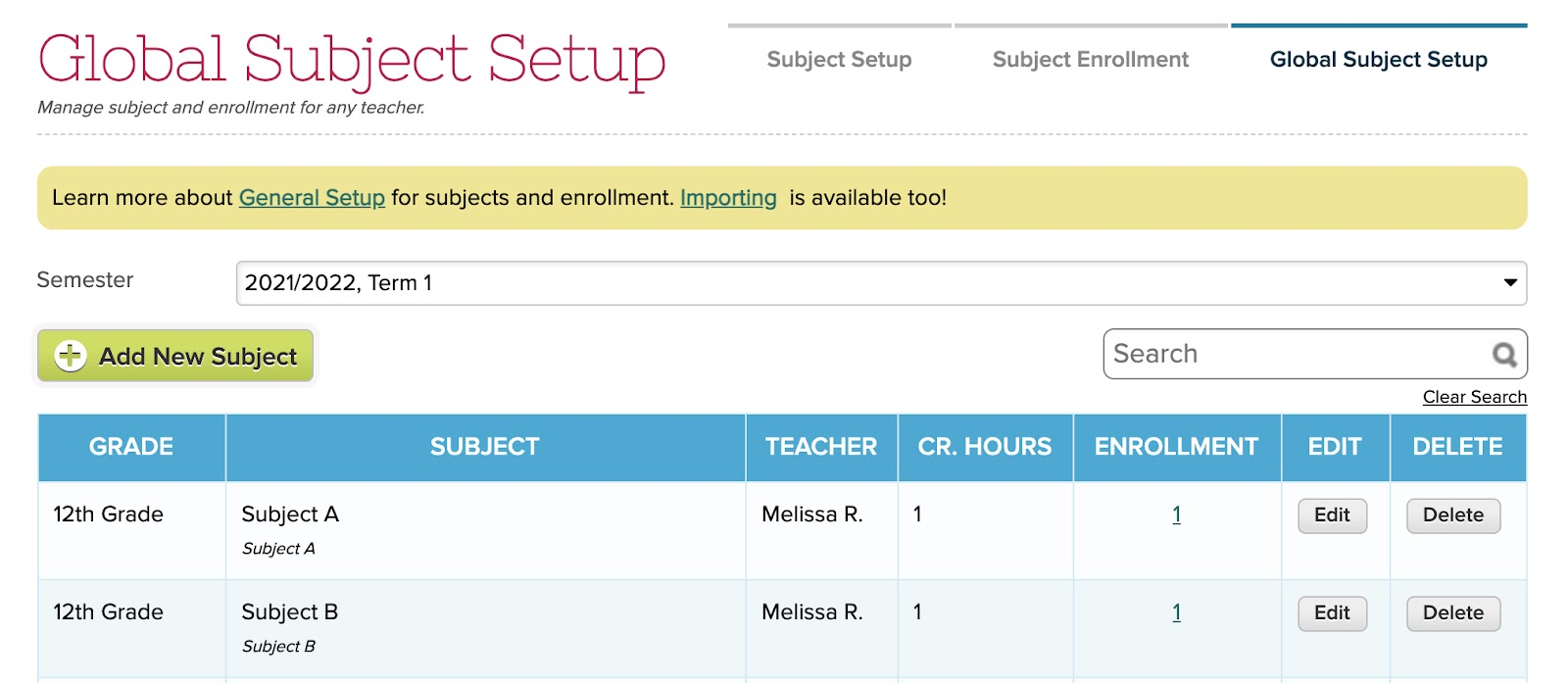#### GPA Table

The GPA Table is where you can set up the point equivalent per letter grade. This is already pre-filled with support for plus and minus letter grades but can easily be edited to match your school’s grading system. The table can be accessed via Transcript > Transcripts Configuration.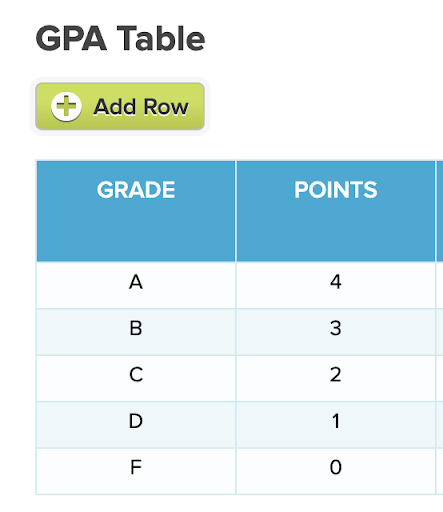### Calculating the GPA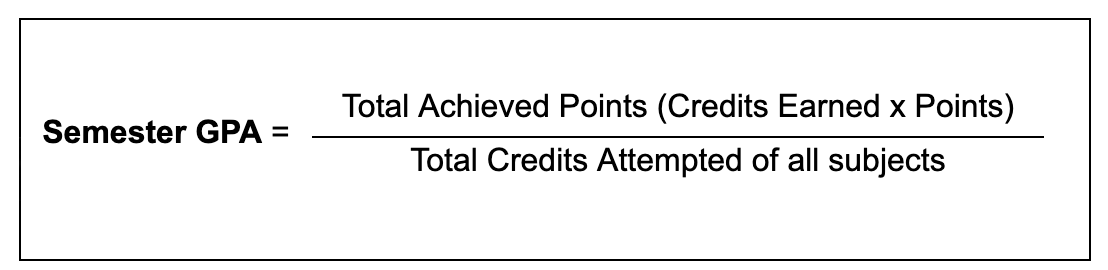For example, a student received a letter grade of A in English, B in Math, F in Science, and an Incomplete for Social Studies, with credits of 0.5 per subject. Based on the table above, A is equivalent to 4 points, B is 3 and F is 0.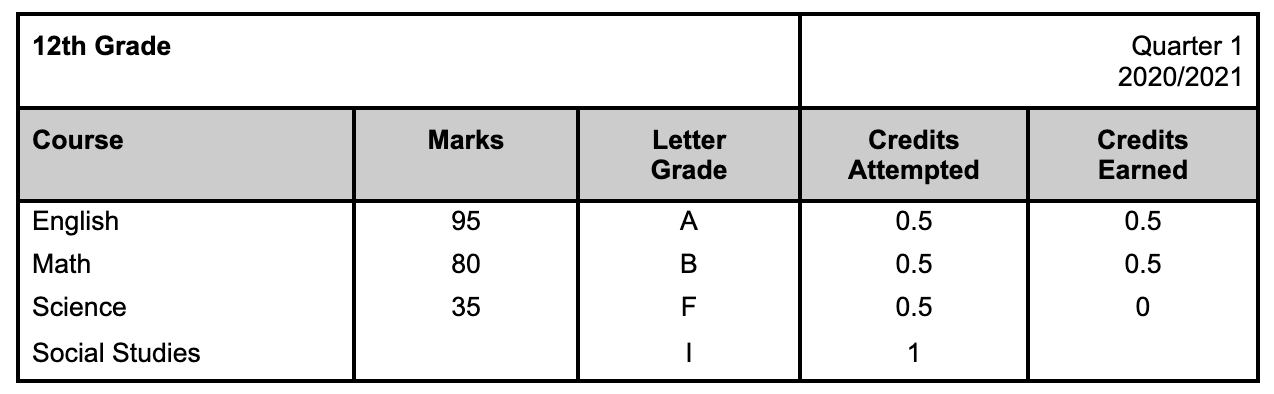First, calculate the achieved points for each subject by multiplying the credits earned to the equivalent point of the letter grade.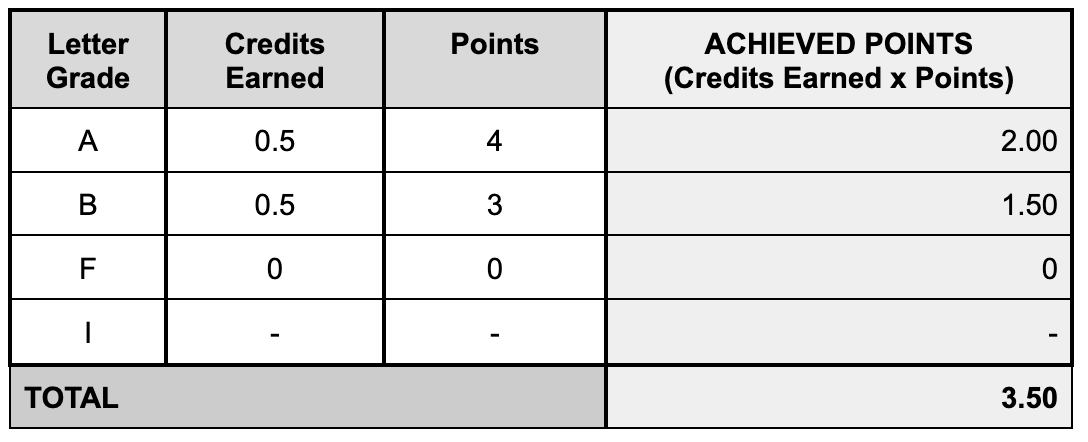Get the total attempted credit hours. If the student failed the subject, the credit hour is still counted while if the student got an “I” or Incomplete, it is excluded.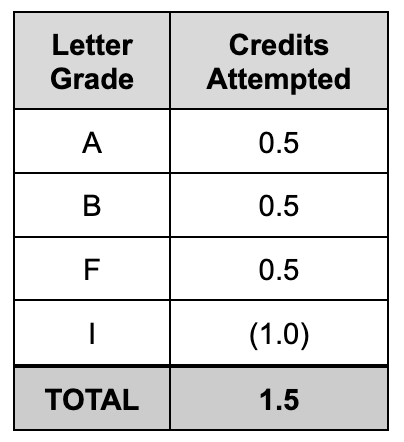Divide the total grade points by total credits attempted to get the GPA for Quarter 1.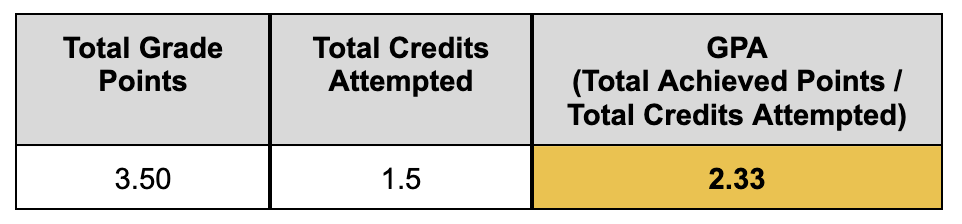### Excluding a subject from GPA calculation

Subjects with 0 credit hours will automatically be excluded from the calculation. However, if you want to give credit hours but are excluded from the GPA calculation, you will need a custom transcript template with custom calculations. The subject will need to contain a code or symbol like an asterisk (*) to remove it from the calculation.

### Weighted GPA

For weighted GPA where classes for Honors, Advanced Placement (AP), or Dual Credit have an additional 0.5 or 1 point equivalent to a letter grade, the subject name must contain the following codes:

• Honors: Honors, (HON)
• AP: AP, (AP), Advanced Placement
• Dual Credit: Dual Credit, (DC)

Using the same example above but with English as honors subject with additional 0.5 points, the weighted GPA would be 2.50.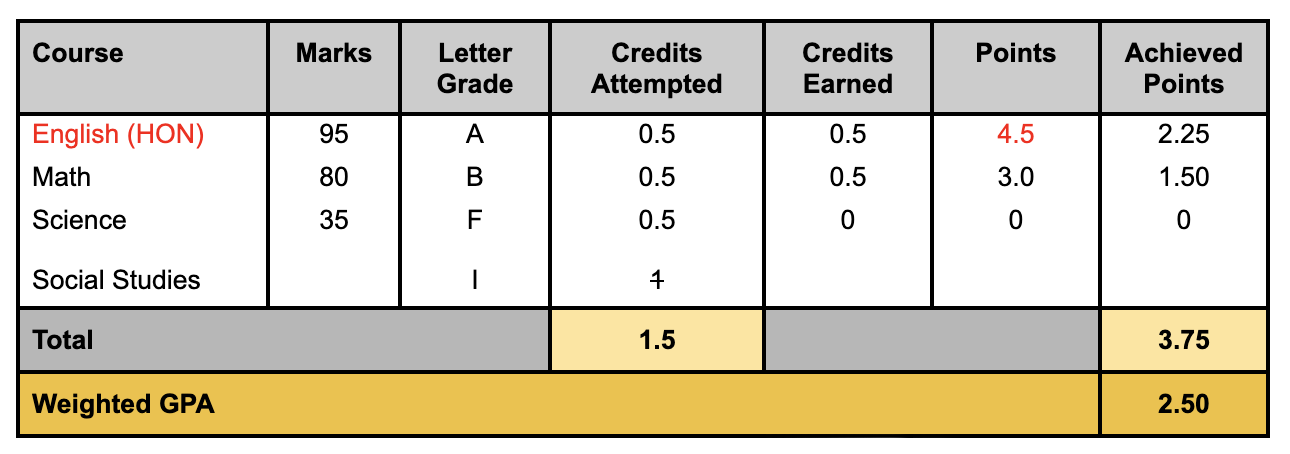To set up the honors points, this must be configured in the template under the Weighted GPA section.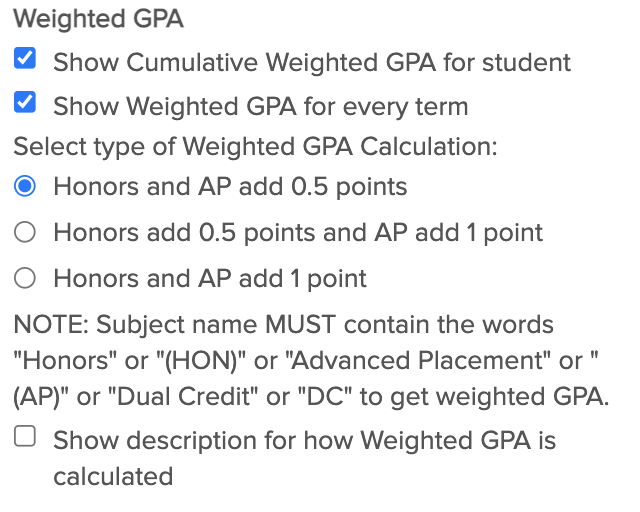Note: This setup for the weighted GPA is only applicable to old public templates. Recently, we have released new transcript templates that support Honors points by simply updating the Honors column in the GPA Table.

### Calculating the Cumulative GPA

The formula for calculating the cumulative GPA is the same as the semester GPA. You’ll have to calculate everything together, not the average of each semester’s GPA.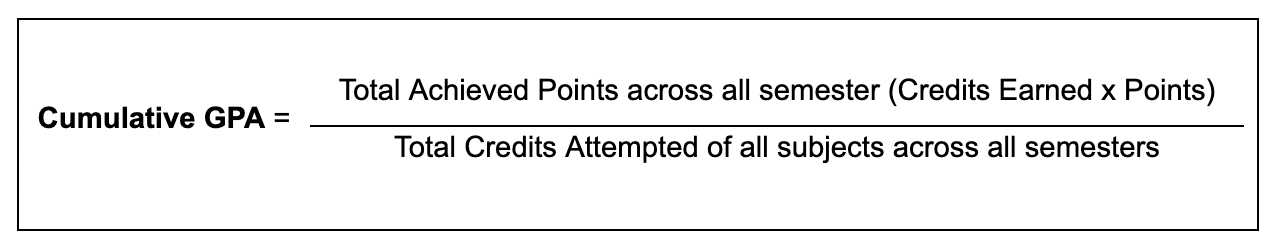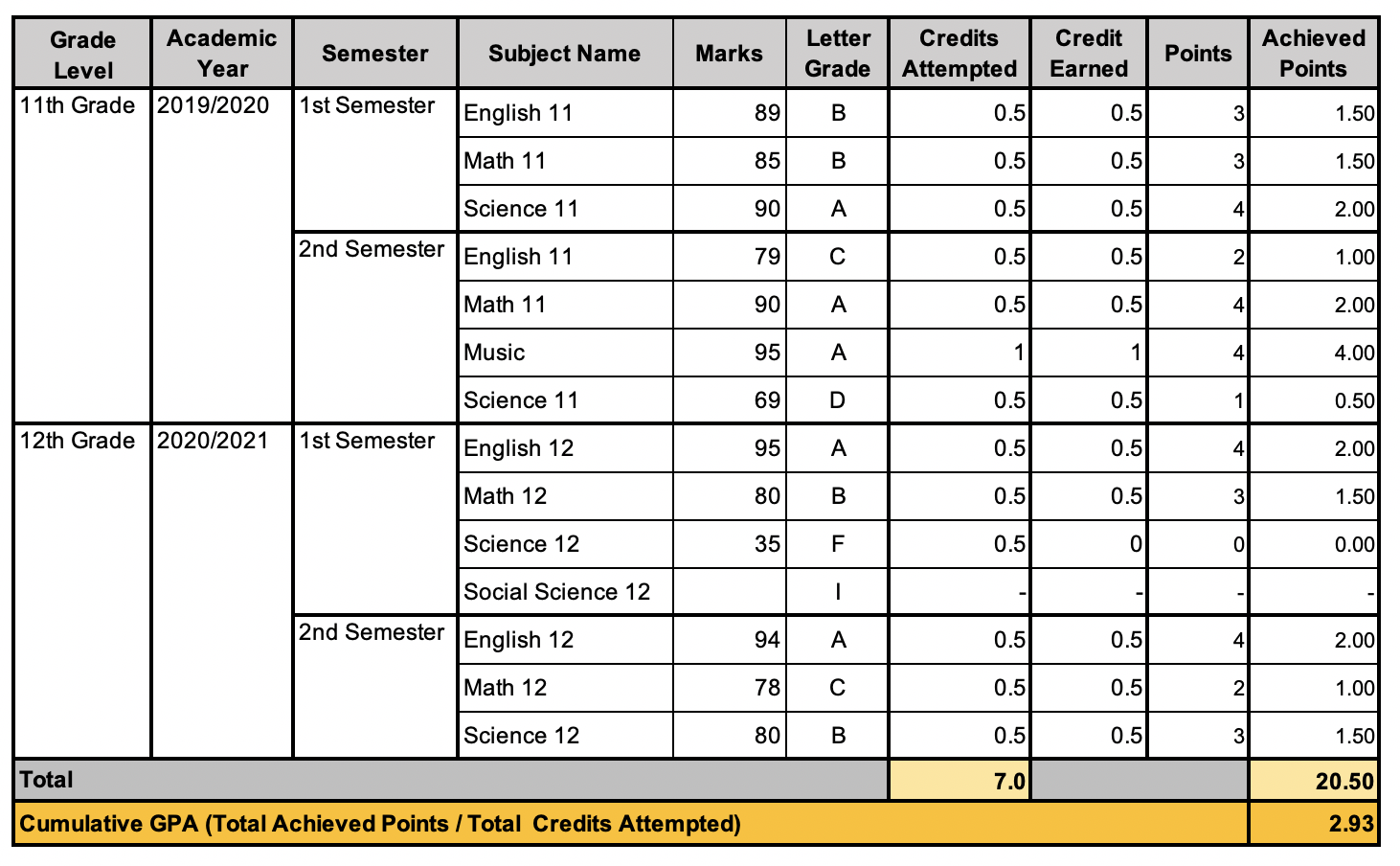The same formula applies to Weighted Cumulative GPA, but with additional points for honors subjects.

Notes:

• It is important to show the letter grades in order to calculate the GPA. If you want the transcripts to show numeric marks only, you would need to temporarily enable the letter grades first then disable them before printing or sending them to students/parents.
• For manually entered subjects and grades, if the GPA is not calculating correctly, check if the Credits Attempted column is showing. Both Credits Attempted and Credits Earned columns must have a value.